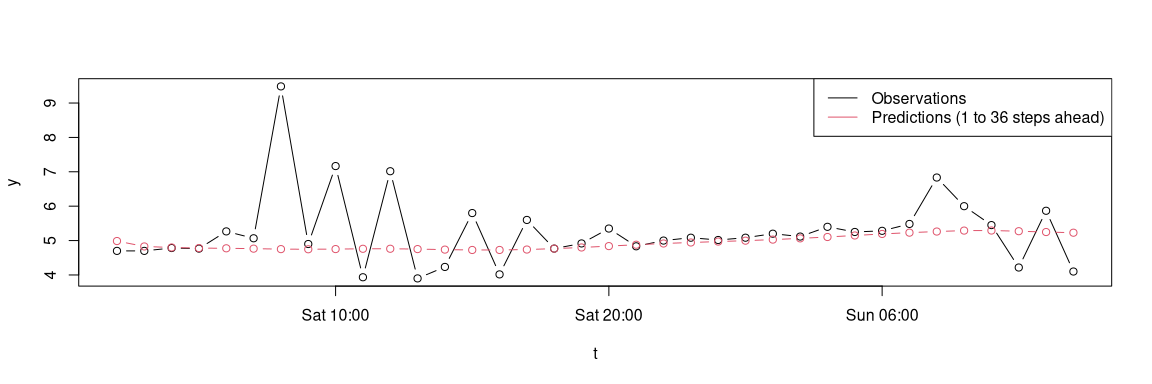# Online updating of onlineforecast models

## Intro

This vignette explains how to use the package in a real online operation, where the following is repeated in real time: new data is received, model is updated and forecasts are calculated. At a lower frequency the parameters are optimized, e.g. the updating is executed every hour and the parameters are optimized once a week.

# Load the package
library(onlineforecast)

Load data, setup and define a model:

# Keep the data in D to simplify notation
D <- Dbuilding
# Set the score period
D$scoreperiod <- in_range("2010-12-20", D$t)
# Set the training period
D$trainperiod <- in_range(D$t, D$t, "2011-02-01") # Define a new model with low-pass filtering of the Ta input model <- forecastmodel$new()
model$output = "heatload" model$add_inputs(Ta = "lp(Ta, a1=0.9)",
mu = "one()")
model$add_regprm("rls_prm(lambda=0.9)") model$add_prmbounds(Ta__a1 = c(0.5, 0.9, 0.9999),
lambda = c(0.9, 0.99, 0.9999))
model$kseq <- 1:36 # Optimize the parameters on the train period (i.e. until 2011-02-01) rls_optim(model, subset(D,D$trainperiod), kseq = c(3,18))
##     ----------------
##     Ta__a1 lambda
##       0.90   0.99
##        k3   k18   sum
##     0.692 0.687 1.378
##     ----------------
##     Ta__a1 lambda
##      0.901  0.990
##        k3   k18   sum
##     0.692 0.687 1.378

## ...output cropped

## Recursive update and prediction

Here we go through the steps of getting new data, running a model update and making predictions.

First fit on a period:

# Keep a sequence with these points
iseq <- which(in_range("2010-12-15",D$t,"2011-01-01")) # Fit the model rls_fit(model$prm, model, subset(D, iseq))
##     ----------------
##     Ta__a1 lambda
##      0.920  0.996

Now the fits are saved in the model object (its an R6 object, hence passed by reference to the functions and can be changed inside the functions). A list of fits with an entry for each horizon is in Lfits, see the two first:

# The data of a fit is saved in this list
str(model$Lfits[1:2]) ## List of 2 ##$ k1:List of 4
##       ..$k : num 1 ## ..$ theta: num [1:2, 1] -0.189 5.134
##       ..$P : num [1:2, 1:2] 0.00118 0.00579 0.00579 0.03359 ## .. ..- attr(*, "dimnames")=List of 2 ## .. .. ..$ : NULL
##       .. .. ..$: chr [1:2] "Ta" "mu" ## ..$ yhat : num [1:36, 1] 6.59 6.57 6.54 6.52 6.5 ...
##      $k2:List of 4 ## ..$ k    : num 2
##       ..$theta: num [1:2, 1] -0.202 5.058 ## ..$ P    : num [1:2, 1:2] 0.00128 0.00634 0.00634 0.03664
##       .. ..- attr(*, "dimnames")=List of 2
##       .. .. ..$: NULL ## .. .. ..$ : chr [1:2] "Ta" "mu"
##       ..$yhat : num [1:36, 1] 6.54 6.51 6.47 6.45 6.42 ... We step to the next time step, where new data arrives. Take the point right after the fit period and take the data for this time point: # Next time step (i <- iseq[length(iseq)] + 1) ##  409 # The new data for this time step Dnew <- subset(D, i) To update and predict, we need to transform the new data (this must only be done only once for each new data, since some transform functions, e.g. lp(), actually keep state data, see some on this in and under ): # Transform the new data DnewTransformed <- model$transform_data(Dnew)

Then we can update the regression coefficients using the transformed data

# The value of the coefficients for horizon 1, before the update
model$Lfits$k1$theta ## [,1] ## [1,] -0.189 ## [2,] 5.134 # Update the coefficients rls_update(model, DnewTransformed, Dnew[[model$output]])
# The value of the coefficients for horizon 1, after the update
model$Lfits$k1$theta ## [,1] ## [1,] -0.19 ## [2,] 5.13 Calculate predictions using the new data and the updated fits: # Calculate the predictions yhat <- rls_predict(model, DnewTransformed) Plot to see the predictions with the observations: # The index for the predicted steps ahead iseq <- i+model$kseq
# Plot the observations and predictions
plot(D$t[iseq], D$heatload[iseq], type = "b", xlab = "t", ylab = "y")
lines(D$t[iseq], yhat, type = "b", col = 2) legend("topright", c("Observations",pst("Predictions (",min(model$kseq)," to ",max(model$kseq)," steps ahead)")), lty = 1, col = 1:2)Run this for a longer period to verify that the same forecasts are obtained (in one go vs. iteratively) First in one go on all data: # Fit and predict on entire data val <- rls_fit(model$prm, model, D)
##     ----------------
##     Ta__a1 lambda
##      0.920  0.996
# Keep the forecasts
D$Yhat1 <- val$Yhat

and then run iteratively through:

# The indexes of training period
itrain <- which(in_range("2010-12-15",D$t,"2011-01-01")) # The indexes of the test period itest <- which(in_range("2011-01-01",D$t,"2011-01-04"))

# Fit on the training period
rls_fit(model$prm, model, subset(D, itrain)) ## ---------------- ## Ta__a1 lambda ## 0.920 0.996 # Prepare for the forecasts D$Yhat2 <- data.frame(matrix(NA, nrow(D$Yhat1), ncol(D$Yhat1)))
names(D$Yhat2) <- names(D$Yhat1)

# Step through the test period:
for(i in itest){
Dnew <- subset(D, i)
Dnewtr <- model$transform_data(Dnew) rls_update(model, Dnewtr, Dnew[[model$output]])
D$Yhat2[i, ] <- as.numeric(rls_predict(model, Dnewtr)) } Compare to see the difference between the one step forecasts: D$Yhat1$k1[itest] - D$Yhat2\$k1[itest]
##       0 0 0 0 0 0 0 0 0 0 0 0 0 0 0 0 0 0 0 0 0 0 0 0 0 0 0 0 0 0 0 0 0 0 0 0 0 0
##      0 0 0 0 0 0 0 0 0 0 0 0 0 0 0 0 0 0 0 0 0 0 0 0 0 0 0 0 0 0 0 0 0 0